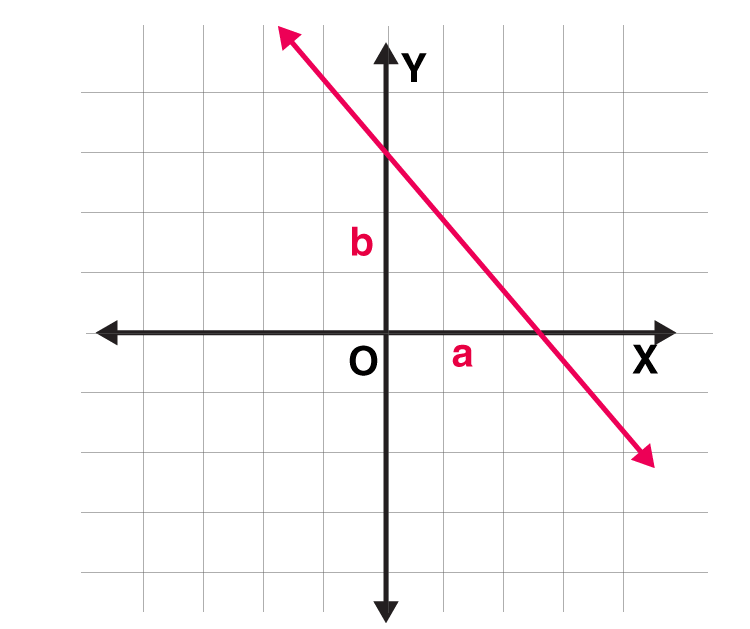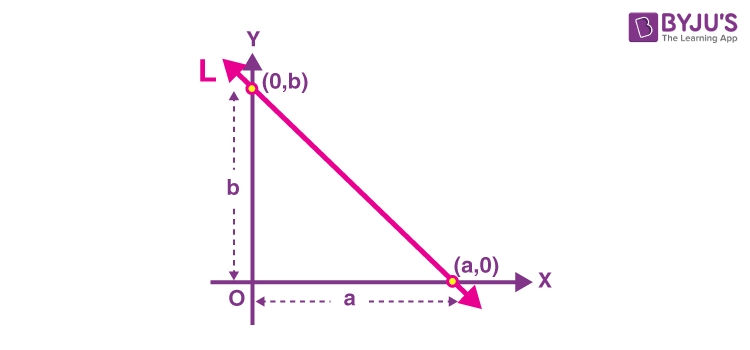# Intercept

In Maths, an intercept is a point on the y-axis, through which the slope of the line passes. It is the y-coordinate of a point where a straight line or a curve intersects the y-axis. This is represented when we write the equation for a line, y = mx+c, where m is slope and c is the y-intercept.

There are basically two intercepts, x-intercept and y-intercept. The point where the line crosses the x-axis is the x-intercept and the point where the line crosses the y-axis is the y-intercept. In this article, you will learn what is the intercept, how to find the intercept for a given line, graphing intercepts along with solved examples.

## Definition of Intercept

The point where the line or curve crosses the axis of the graph is called intercept. If a point crosses the x-axis, then it is called the x-intercept. If a point crosses the y-axis, then it is called the y-intercept.

The meaning of intercept of a line is the point at which it intersects either the x-axis or y-axis. If the axis is not specified, usually the y-axis is considered. It is normally denoted by the letter ‘b’.

Except that line is accurately vertical, it will constantly cross the y-axis somewhere, even if it is way off the top or bottom of the chart.

Also read: Equation of plane in intercept form

## Intercept Formula

The equation of the line, which intersects the y-axis at a point is given by:
y = mx + c
Now, we have to write the intercept form of the line, we can replace c with b. Thus, the equation becomes:

y = mx + b
Hence, the formula for the y-intercept of a line is given by:
b = y – mx
Where, b is the intercept, m is the slope of the line and y and x indicate the points on the y-axis and x-axis respectively.

Now, another way of writing the equation of the line, considering a line is intersecting the x-axis and y-axis at point a and b respectively.
x/a + y/b = 1
Here, a and b are the intercepts of the line which intersect the x-axis and y-axis, respectively. The values of a and b can be positive, negative or zero and explain the position of the points at which the line cuts both axes, relative to the origin.

## How to Find X and Y Intercepts?

Consider a straight line equation Ax + By = C.

Divide the equation by C,

(Ax/C) + (By/C) = C/C

[x/(C/A)] + [y/(C/B)] = 1

Comparing this equation with the equation of a line in intercept form, (x/a) + (y/b) = 1,

We get, x-intercept = a = C/A

y-intercept = b = B/C

Alternatively,

To find the x-intercept, substitute y = 0 and solve for x.

i.e. Ax + B(0) = C

Ax = C

x = C/A

To find the y-intercept, substitute x =0 and solve for y.

i.e. A(0) + By = C

By = C

y = C/B

Go through the example given below to understand this concept in a better way.

Example: Let us assume the straight-line equation 5x +2y =10

To find x-intercept:

Substitute y=0 in the given equation

5x + 2(0) = 10

5x =10

x =2

To find y-intercept

Substitute x =0 in the given equation

5(0) + 2y =10

2y = 10

y = 5

Therefore, x -intercept is (2, 0)

y -intercept is (0, 5)

## Two Point Form

The formula of the line formed by the two points is given by:

y-y1/y2-y1 = x-x1/x2-x1Say, P(a, 0) = (x1, y1) and Q(0, b) = (x2, y2) are the two points of the line which cuts the x-axis and y-axis, relative to the origin(0,0). Then the formula becomes:

=> y – 0 / b – 0 = x – a/ 0 – a

=> y/b = x/-a – a/-a

=> x/a + y/b = 1

Hence, proved.

## Slope Intercept Form

The equation of the line making an intercept c on the y-axis and having slope m is given by:

y = mx + c

Note: The value of c could be positive or negative since the intercept is drawn on the positive or negative side of the y-axis, respectively.

Also, check: Slope intercept form

## Intercept Graph

The intercepts are the points on a graph at which the graph crosses the two axes (x-axis and y-axis). The point where the graph crosses the x-axis is called the x-coordinate and the point where the graph crosses the y-axis is called the y-coordinate.In the above intercept graph, where a line L makes x-intercept a and y-intercept b on the axes.

Thus, the equation of the line making intercepts a and b on the x-and y-axis, respectively, is:

x/a + y/b = 1

## Solved Examples

Example 1: Let two intercepts P(2,0) and Q(0,3) intersect the x-axis and y-axis, respectively. Find the equation of the line.

Solution: Given, two intercepts P(2,0) and Q(0,3) intersect the x-axis and y-axis.

From the equation of the line we know,

x/a + y/b = 1 ……….. (1)

Here, a = 2 and b = 3

Therefore, putting the values of intercepts a and b, in equation 1, we get:

=>x/2 + y/3 = 1

=> 3x + 2y = 6

=> 3x + 2y – 6 = 0,

Therefore, the equation of the line is 3x + 2y – 6 = 0.

Example 2: Find the equation of the line, which makes intercepts –3 and 2 on the x- and y-axes respectively.

Solution: Given, a = –3 and b = 2.

By intercept form, we know that;

x/a + y/b = 1

x/-3 + y/2 = 1

Or

2x – 3y + 6 = 0.

Hence, this is the required equation.

Example 3: A line passes through P (1, 2) such that its intercept between the axes is bisected at P. What is the equation of the line?

Solution: The equation of a line making intercepts a and b with x-axis and y-axis, respectively, is given by:

x/a + y/b = 1

1 = (a+0)/2 ⇒ a = 2

2 = (0 + b)/2 ⇒ b = 4

Therefore, the required equation of line is;

x/2 + y/4 = 1

⇒ 2x + y – 4 = 0

### Practice Problems

1. Find the x-intercept and y-intercept for the line 5x – 8y = 2.
2. If the y-intercept of a line is -4 and the slope is 2/3, then write its equation.
3. What is the equation of a line whose x and y-intercepts are given as 1/3 and -3?

Stay tuned with BYJU’S – The Learning App and download the app to explore more videos.

## Frequently Asked Questions – FAQs

### What is an intercept in Maths?

An intercept is a point where the straight line or a curve intersects the y-axis in a plane. It is also said to be a y-intercept.

### What is the formula for y-intercept?

The formula for y-intercept is given by:
b = y – mx
Where b is the y-intercept and m is the slope of the line

### What is the equation of the line with respect to x and y-intercepts?

The equation of the line, when a line is intersecting the x-axis and y-axis at point a and b respectively is given by:
x/a + y/b = 1

### If a line intersects the y-axis at a point, then what is the value of x-coordinate?

If a line intersects the y-axis at a point, the value of the x-coordinate will be equal to zero.

### What is the slope formula?

m=(y2-y1)/(x2-x1)

Quiz on Intercept